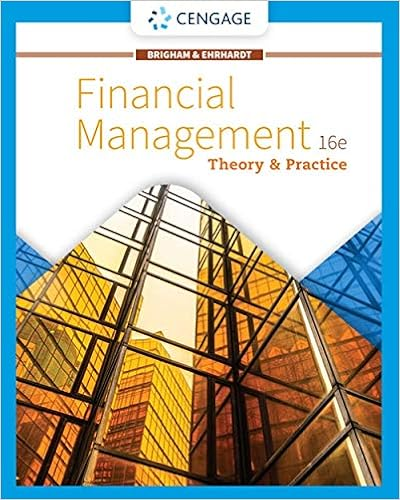# If the hedge ratio for a stock call is 060 the hedge

• Notes
• 84
• 95% (21) 20 out of 21 people found this document helpful

This preview shows page 60 - 65 out of 84 pages.

##### We have textbook solutions for you!
The document you are viewing contains questions related to this textbook.The document you are viewing contains questions related to this textbook.
Chapter 8 / Exercise 8-3
Financial Management: Theory & Practice
Brigham/EhrhardtExpert Verified
50.If the hedge ratio for a stock call is 0.60, the hedgeratio for a put with the same expiration date and exercise price as the call would be A. 0.6
##### We have textbook solutions for you!
The document you are viewing contains questions related to this textbook.The document you are viewing contains questions related to this textbook.
Chapter 8 / Exercise 8-3
Financial Management: Theory & Practice
Brigham/EhrhardtExpert Verified
0.B. 0.40.C. -0.60.D.-0.40.E. -.17.Call hedge ratio = N(d1); Put hedge ratio = N(d1) -1; 0.6 - 1.0 = -0.4.AACSB: AnalyticBlooms: ApplyDifficulty: ChallengeTopic: Option Valuation51.If the hedge ratio for a stock call is 0.70, the hedgeratio for a put with the same expiration date and exercise price as the call would be A. 0.70.B. 0.30.C. -0.70.D.-0.30.E. -.17.Call hedge ratio = N(d1); Put hedge ratio = N(d1) -1; 0.7 - 1.0 = -0.3.AACSB: AnalyticBlooms: ApplyDifficulty: ChallengeTopic: Option Valuation52.A put option is currently selling for \$6 with an exercise price of \$50. If the hedge ratio for the put is -0.30 and the stock is currently selling for \$46, what is the elasticity of the put? 21-61
A. 2.76B. 2.30C. -7.67D. -2.76E.-2.30% stock price change = (\$47 - \$46)/\$46 = 0.021739; % option price change = (\$5.70 - \$6.00)/\$6 = - 0.05; - 0.05/0.021739 = - 2.30.AACSB: AnalyticBlooms: ApplyDifficulty: ChallengeTopic: Option Valuation53.A put option on the S&P 500 Index will best protecta portfolio A. of 100 shares of IBM stock.B. of 50 bonds.C.that corresponds to the S&P 500.D. of 50 shares of AT&T and 50 shares of Xerox stocks.E. that replicates the Dow.The S&P 500 Index is more like a portfolio that corresponds to the S&P 500 and thus is more protective of such a portfolio than of any of the other assets.AACSB: AnalyticBlooms: ApplyDifficulty: BasicTopic: Option Valuation54.Higher dividend payout policies have a __________ impact on the value of the call and a __________ impact on the value of the put
compared to lower dividend payout policies. A. negative; negativeB. positive; positiveC. positive; negativeD.negative; positiveE. zero; zeroDividends lower the expected stock price, and thuslower the current call option value and increase the current put option value.AACSB: AnalyticBlooms: ApplyDifficulty: IntermediateTopic: Option Valuation55.Lower dividend payout policies have a __________ impact on the value of the call and a __________ impact on the value of the put compared to higher dividend payout policies. A. negative; negativeB. positive; positiveC.positive; negativeD. negative; positiveE. zero; zeroDividends lower the expected stock price, and thuslower the current call option value and increase the current put option value.AACSB: AnalyticBlooms: ApplyDifficulty: IntermediateTopic: Option Valuation21-63
56.A \$1 decrease in a call option's exercise price would result in a(n) __________ in the call option'svalue of __________ one dollar. A. increase; more thanB. decrease; more thanC. decrease; less thanD.increase; less thanE. increase; exactlyOption prices are less than stock prices, thus changes in stock prices (market or exercise) are greater (in absolute terms) than are changes in prices of options.AACSB: AnalyticBlooms: UnderstandDifficulty: IntermediateTopic: Option Valuation
•••